## 前言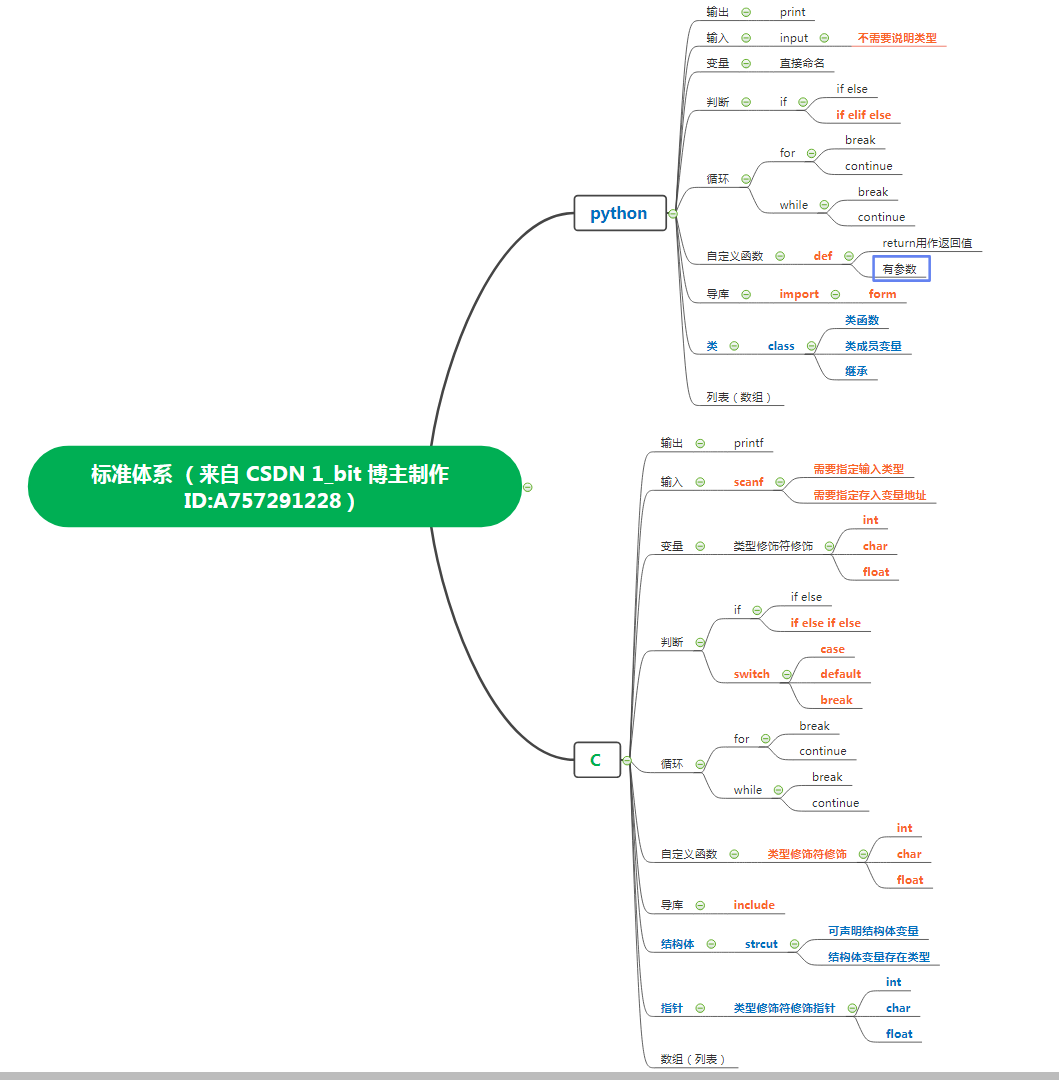# 欢迎关注我哟~4/14日我还准备开播解答新手问题哟 。有问题可以私聊我，我是一个热心博主。

## 一 输出

C：

``````printf("HelloWorld");
``````

Python：

``````print("HelloWorld")
``````

C 与 Python 之中的输出显示分别使用 printf 与 print，其实较为完整的写法应该是在 printf 或 print 后添加一堆圆括号（在编程中所有有功能作用的标点都必须使用英文），printf 与 print 我们称为函数名 ，我们使用这个函数就直接使用函数名加圆括号即可，圆括号中的内容我们可以称为参数，参数可以理解为做饭时使用拥有做饭功能的电饭锅所加入的米 ，因为有米才能做饭，在这里有需要显示内容则有指定内容才能显示 。在使用 printf 与 print 时，我们在这两个函数的圆括号中传入了我们需要显示的内容 ，在程序运行时将会显示其内容。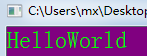## 二 变量

``````int a=0;
``````

``````a=0
``````

### 变量输出

C语言使用 printf 进行变量的内容输出对比 python 较为繁琐，示例如下：

``````int a=0;
printf("%d",a);
``````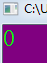``````int a=0;
int b=10;
printf("%d %d ",a,b);
``````

``````a=10
b=0
print(a,b)
``````

### C语言变量类型

``````int a=0;
char b='A';
float tt=3.33;
printf("%d %c %f ",a,b,tt);
``````

## 三 输入

``````int a=0;
scanf("%d",&a);
``````

``````b=0
b=input()
``````

input 是 python 语言中接收用户输入的函数 ，直接将 input 函数赋值到一个变量即可；此时运行到这一句代码时，input 函数发生作用，等待用户输入一个值确认后存储至 b 变量之中 。

## 四 判断

``````int a=0;
scanf("%d",&a);
if(a==3){
printf("用户输入的是 3 ");
}
``````

if 是关键字 ，我们可以理解关键字为有特殊功能的字符，在这里 if 就是表示接下来需要进行判断了 。在 if 关键字后的圆括号中，a==3 表示判断 a 是否等于3.在 C 语言中 ，使用两个等于号表示判断等于号左右两边的值是否相等；此处，如果该条件成立，那么将会执行圆括号后的花括号内的代码 ，也就是显示 用户输入的是 3 ，如果条件错误，用户输入的值不是 3 将会什么都不显示。

``````a=int(input())
if a==3:
print("用户输入的是 3 ")
``````

``````a=int(input())
if a==3:
print("用户输入的是 3 ")
print("用户输入的是 3 ")
print("用户输入的是 3 ")
``````

### if else

C语言例：

``````int a=0;
scanf("%d",&a);
if(a==3){
printf("用户输入的是 3 ");
}else{
printf("用户输入的不是 3");
}
``````

Python 语言例：

``````a=int(input())
if a==3:
print("用户输入的是 3 ")
else:
print("用户输入的不是 3 ")
``````

### if else if

``````int a=0;
scanf("%d",&a);
if(a==3){
printf("用户输入的是 3 ");
}else if(a==11){
printf("用户输入的不是 11");
}else if(a==5){
printf("用户输入的不是 5");
}else{
printf("用户输入是其他数");
}
``````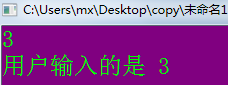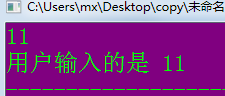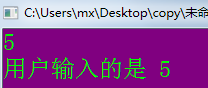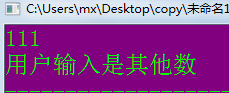``````a=int(input())
if a==3:
print("用户输入的是 3 ")
elif a==11:
print("用户输入的是 11")
elif a==5:
print("用户输入的是 5")
else:
print("用户输入是其他数")
``````

## 五 循环

### while 循环

C 语言的 while 循环：

``````int a=0;
while(a<10){
printf("我在循环...");
a++;
}
``````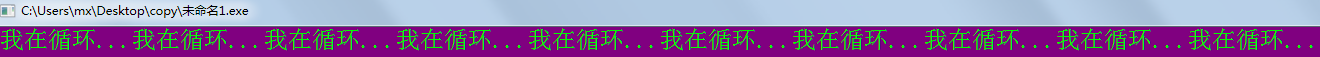``````a=0
while a<10:
print("我在循环...")
a+=1
``````

### for 循环

for 循环在 C 语言与 Python 语言中有一些区别，不过大体一致 ，首先我们查看 C 语言的 for 循环代码：

``````int a;
for(a=0;a<10;a++){
printf("我在循环...");
}
``````

Python 语言的 for 循环代码如下：

``````for i in range(10):
print("我在循环")
``````

### break 与 continue

break 与 continue 均是用于循环控制 ，若我想循环到第五次时就不再循环可以使用 break。

C语言示例：

``````int a;
for(a=0;a<10;a++){
if(a==5){
break;
}
printf("我在循环...");
}
``````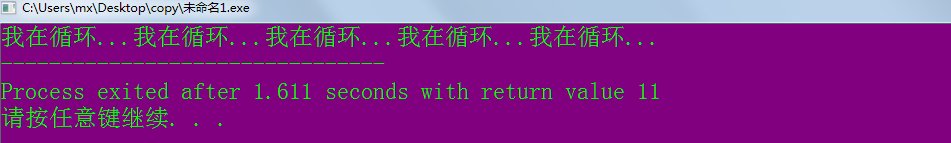Python 中此功能实现也是使用break，代码如下：

``````for i in range(10):
if i==5:
break
print("我在循环")
``````

## 六 自定义函数

``````#include<stdio.h>
int r1(){
return 1;
}

int main(){
int a;
a=r1();
printf("%d ",a);
}
``````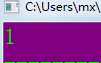``````#include<stdio.h>

int r1(int a,int b){
return a+b;
}

int main(){
int a;
a=r1(11,2);
printf("%d ",a);
}
``````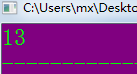Python 中的自定义相比 C 语言简单了不少 ，不需要说明函数返回值类型，参数也不需要标识类型；我们实现同一个功能只需简单的几行代码：

``````def r1(a,b):
return a+b

a=r1(11,2)
print(a)
``````

## 七 导库

C语言的#include 不再进行说明 ，因为基础代码中就有其存在，我们讲解 Python 中的 import。在 Python 若想让我们的程序休眠几秒可以使用一个库叫做 time，或者说是时间库 ，我们需要停止时间所使用的停止工具就在这个工具箱中，代码示例如下：

``````import time
def r1(a,b):
return a+b

time.sleep(3)
a=r1(11,2)
print(a)
``````

import time 表示将 time 这个工具箱拿过来，time.sleep 表示使用 time 这个工具箱中的 sleep 工具 ，sleep 作用为停止几秒，在圆括号中输入秒数即可，其中的小数点我们可以理解为“的 ” ，某某工具箱中“的”某某工具 。

## 数组（列表）

C语言中的数组：

``````int a[]={9,6,3,2,5,8,7,4,1,0};
printf("%d ",a);
``````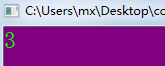Python 中的列表也类似：

``````a=[9,6,3,2,5,8,7,4,1,0]
print(a)
``````